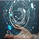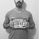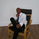# Best Scripts of the Month — 2020.06

Education
NASDAQ:SSP   SCRIPPS (EW) COMPANY
12229 views
We present you with our selection from the open-source indicators published this month on TradingView. These scripts are the ones that caught our attention while analyzing the two thousand or so scripts published each month in TradingView's Public Library, the greatest repository of indicators in the world. If we missed any, please let us know. Most of these scripts were selected on the basis of their potential to be useful in trading; others will be more useful to Pine coders.

This superb body of work constitutes unmistakable proof of the liveliness, ingenuity, and relentless creativity of the generous traders/programmers who not only give their time to write amazing scripts, but share their code with the world. Kudos to these very special humans.

Enjoy!

Time Range Statistics
alexgrover continues to mine time series for all they are worth. This script shows traders some key statistics for a symbol.
═•═•═•═•═•═•═•═•═•═•═•═•═•═•═•═•═•═•═•═•═•═•═•═•═•═•═•═•═•═•═•═•═•═•═•═•═•═•═•═•═•═•═•═

BTC_JackSparrow uses Quandl data to show periodic BTC difficulty adjustments.
═•═•═•═•═•═•═•═•═•═•═•═•═•═•═•═•═•═•═•═•═•═•═•═•═•═•═•═•═•═•═•═•═•═•═•═•═•═•═•═•═•═•═•═

Bayesian BBSMA Oscillator
tista builds a model calculating the probability of an eventual up/down movement.
═•═•═•═•═•═•═•═•═•═•═•═•═•═•═•═•═•═•═•═•═•═•═•═•═•═•═•═•═•═•═•═•═•═•═•═•═•═•═•═•═•═•═•═

Binomial Option Pricing Model
We see more and more scripts dedicated to options trading. SegaRKO presents a method that works on more types of options than the Black-Scholes Model.
═•═•═•═•═•═•═•═•═•═•═•═•═•═•═•═•═•═•═•═•═•═•═•═•═•═•═•═•═•═•═•═•═•═•═•═•═•═•═•═•═•═•═•═

Funamental and financials
sjakk mines a stock's fundamentals using the new `financial()` function and presents them in a usable format.
═•═•═•═•═•═•═•═•═•═•═•═•═•═•═•═•═•═•═•═•═•═•═•═•═•═•═•═•═•═•═•═•═•═•═•═•═•═•═•═•═•═•═•═

VAMA Volume Adjusted Moving Average Function
A volume-adjusted moving average calculated using a technique by Richard Arm, brought to us by allanster.
═•═•═•═•═•═•═•═•═•═•═•═•═•═•═•═•═•═•═•═•═•═•═•═•═•═•═•═•═•═•═•═•═•═•═•═•═•═•═•═•═•═•═•═

Hull Candles
Indicator designers are just beginning to scratch the surface of price representation on charts. BigBitsIO takes us further on that path with this well-designed take on candles using a very appropriate Hull MA to build and color them.
═•═•═•═•═•═•═•═•═•═•═•═•═•═•═•═•═•═•═•═•═•═•═•═•═•═•═•═•═•═•═•═•═•═•═•═•═•═•═•═•═•═•═•═

Support Resistance MTF
LonesomeTheBlue provides S/R levels from a user-selected timeframe without using `security()` and displays them in a useful way.
═•═•═•═•═•═•═•═•═•═•═•═•═•═•═•═•═•═•═•═•═•═•═•═•═•═•═•═•═•═•═•═•═•═•═•═•═•═•═•═•═•═•═•═

SwingArm ATR Trend Indicator
vsnfnd provides a trend-following trading system based on ATR.
═•═•═•═•═•═•═•═•═•═•═•═•═•═•═•═•═•═•═•═•═•═•═•═•═•═•═•═•═•═•═•═•═•═•═•═•═•═•═•═•═•═•═•═

Trend Quality
kruskakli's script uses MA states and their relative positions to derive an interesting take on the current state of the trend.
═•═•═•═•═•═•═•═•═•═•═•═•═•═•═•═•═•═•═•═•═•═•═•═•═•═•═•═•═•═•═•═•═•═•═•═•═•═•═•═•═•═•═•═

Stochastic Weights - Basic
BigBitsIO introduces a Stoch indicator allowing the signal to be composed of weighed variations of the Stoch signal.
═•═•═•═•═•═•═•═•═•═•═•═•═•═•═•═•═•═•═•═•═•═•═•═•═•═•═•═•═•═•═•═•═•═•═•═•═•═•═•═•═•═•═•═

Donchian HL Width - Cycle Information
RicardoSantos calculates the relationship between high/low and Donchian channels as a percentage, and uses that information to detect cycles.
═•═•═•═•═•═•═•═•═•═•═•═•═•═•═•═•═•═•═•═•═•═•═•═•═•═•═•═•═•═•═•═•═•═•═•═•═•═•═•═•═•═•═•═

TF Segmented Linear Regression
alexgrover allows traders to see periodic linear regressions calculated from a user-selected timeframe.
═•═•═•═•═•═•═•═•═•═•═•═•═•═•═•═•═•═•═•═•═•═•═•═•═•═•═•═•═•═•═•═•═•═•═•═•═•═•═•═•═•═•═•═

█ CODER UTILITIES

Periodic Ellipses
alexgrover comes up with the first Pine code we see that draws ellipses.
═•═•═•═•═•═•═•═•═•═•═•═•═•═•═•═•═•═•═•═•═•═•═•═•═•═•═•═•═•═•═•═•═•═•═•═•═•═•═•═•═•═•═•═
Tools and ideas for all Pine coders: http://www.pinecoders.com
Our Pine FAQ & Code: http://www.pinecoders.com/faq_and_code/